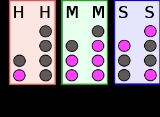xBinary numeral systemOverview

The binary numeral system, or base-2 number system, represents numeric values using two symbols, 0
0 (number)
0 is both a numberand the numerical digit used to represent that number in numerals.It fulfills a central role in mathematics as the additive identity of the integers, real numbers, and many other algebraic structures. As a digit, 0 is used as a placeholder in place value systems...

and 1. More specifically, the usual base-2 system is a positional notation
Positional notation
Positional notation or place-value notation is a method of representing or encoding numbers. Positional notation is distinguished from other notations for its use of the same symbol for the different orders of magnitude...

In mathematical numeral systems, the base or radix for the simplest case is the number of unique digits, including zero, that a positional numeral system uses to represent numbers. For example, for the decimal system the radix is ten, because it uses the ten digits from 0 through 9.In any numeral...

of 2. Because of its straightforward implementation in digital electronic circuitry using logic gate
Logic gate
A logic gate is an idealized or physical device implementing a Boolean function, that is, it performs a logical operation on one or more logic inputs and produces a single logic output. Depending on the context, the term may refer to an ideal logic gate, one that has for instance zero rise time and...

s, the binary system is used internally by almost all modern computer
Computer
A computer is a programmable machine designed to sequentially and automatically carry out a sequence of arithmetic or logical operations. The particular sequence of operations can be changed readily, allowing the computer to solve more than one kind of problem...

s.
The Indian scholar Pingala
Pingala
Pingala is the traditional name of the author of the ' , the earliest known Sanskrit treatise on prosody.Nothing is known about Piṅgala himself...

(circa
Circa
Circa , usually abbreviated c. or ca. , means "approximately" in the English language, usually referring to a date...

5th–2nd centuries BC) developed mathematical concepts for describing prosody, and in so doing presented the first known description of a binary numeral system.